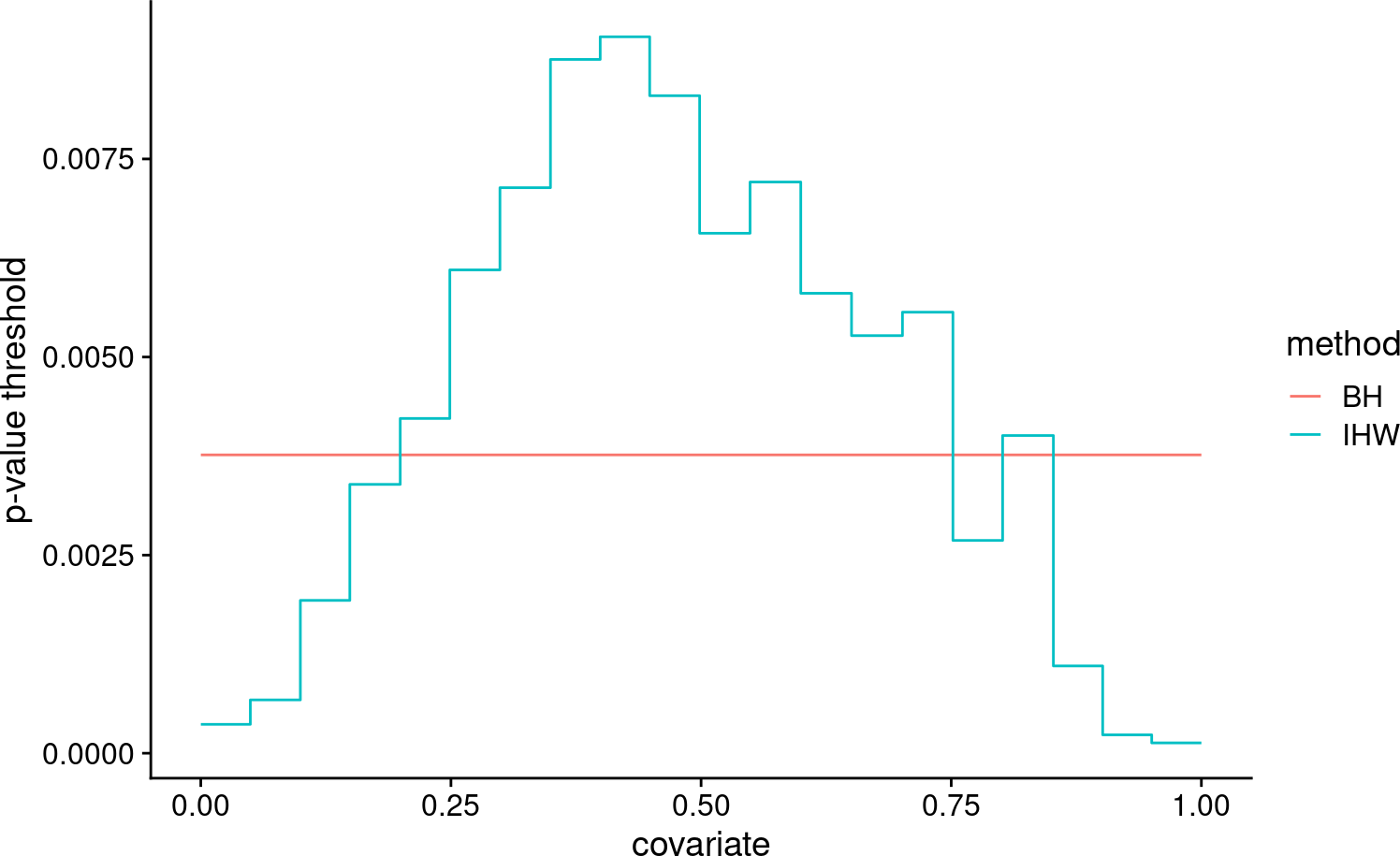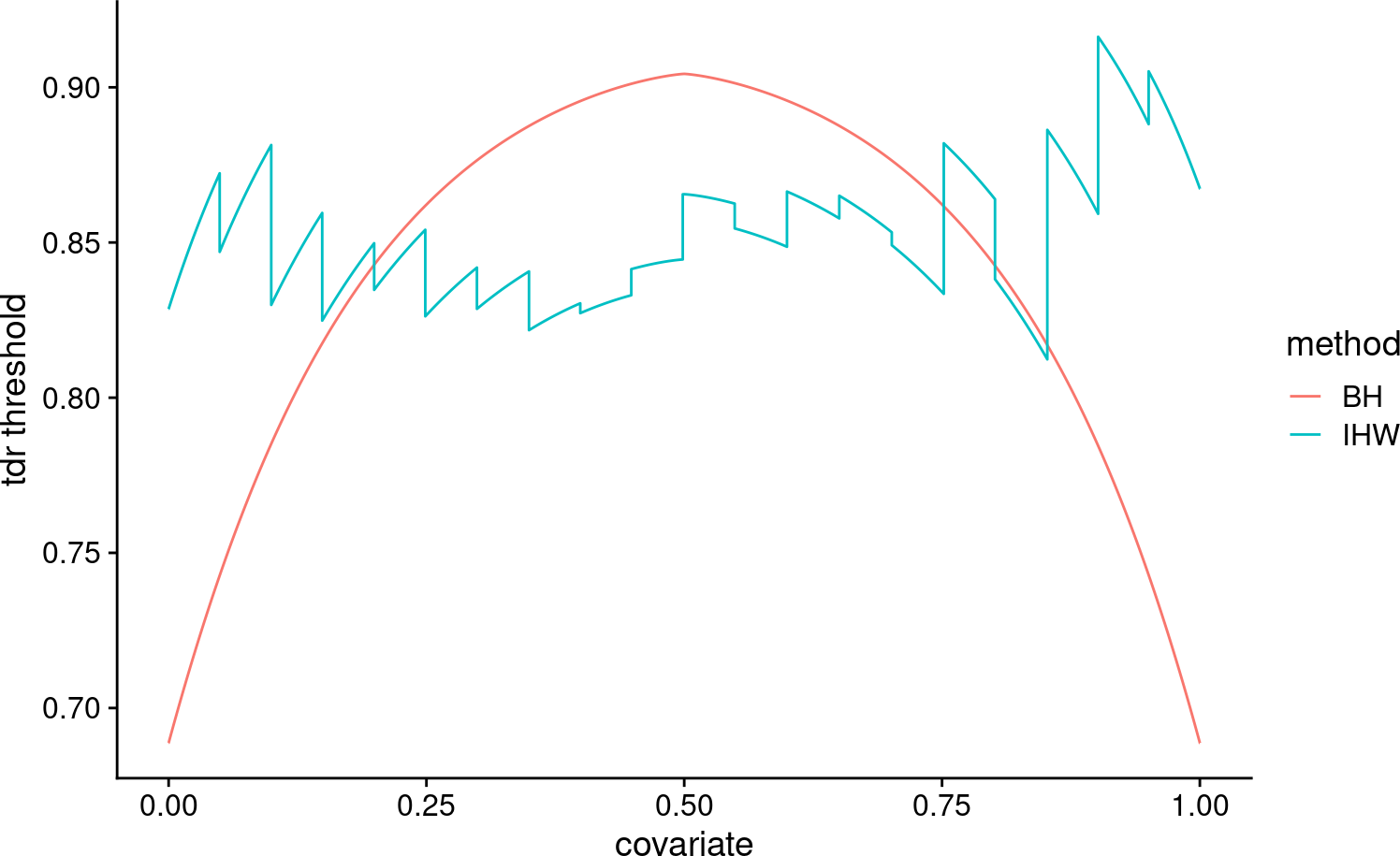One of the differences between BH and IHW is that BH sets a constant rejection threshold across all hypotheses which does not depend on the covariate. The rejection threshold of IHW on the other hand depends on the covariate. In many situations, this means, that the BH rejection threshold will be non-constant as a function of the tdr, while IHW will approximate a constant tdr threshold. Here we want to explore this using a simple simulation.

library("ggplot2")
library("dplyr")
library("tidyr")
library("gridExtra")
#library("LSD")
library("IHW")

We set up the helper function which generates the simulation, applies BH and IHW and evaluates the tdr at the rejection thresholds.

# try function with non-monotonic relation
sim <- function(m =20000){
x <- 1-runif(m)
pi0 <- 0.75+0.3*(x-0.5)^2#0.8 #ifelse(x<=.5, 0.5 + 0.6*x, 0.8)
eff_size <- 2-2.2*abs(x-0.5)^1.3  #was 3.2 #ifelse(x <=.5, 1.5, 1.5-(x-1/2)*4)
H <- rbinom(m, 1, 1-pi0)
Z <- rnorm(m)
Z[H==1] <- rnorm(sum(H), eff_size[H==1])
pvalue <- 1-pnorm(Z)
t <- pvalue
t_bh <- get_bh_threshold(pvalue,0.1)

alt_ft_bh <- dnorm(-eff_size+ qnorm(1-t_bh))/dnorm(qnorm(1-t_bh))
tdr_bh <- alt_ft_bh*(1-pi0)/((1-pi0)*alt_ft_bh + pi0)
t_ddhw <- thresholds(ihw(pvalue, x, alpha= .1, nbins=20,
nsplits_internal=10, nfolds = 1), levels_only=FALSE)

alt_ft_ddhw <- dnorm(-eff_size+ qnorm(1-t_ddhw))/dnorm(qnorm(1-t_ddhw))
tdr_ddhw <- alt_ft_ddhw*(1-pi0)/((1-pi0)*alt_ft_ddhw + pi0)

alt_ft <- dnorm(qnorm(1-t)-eff_size)/dnorm(qnorm(1-t))
tdr <- alt_ft*(1-pi0)/((1-pi0)*alt_ft + pi0)
return(data.frame(x=x,pi0=pi0, t=t, eff_size=eff_size,H=H,
Z=Z, pvalue=pvalue, alt_ft=alt_ft, tdr=tdr,
tdr_bh=tdr_bh, alt_ft_bh=alt_ft_bh, t_bh=t_bh,
t_ddhw=t_ddhw, tdr_ddhw=tdr_ddhw, alt_ft_ddhw=alt_ft_ddhw))
}

Run above simulation:

set.seed(1)
sim_df <- sim(m=80000)
## Using only 1 fold! Only use this if you want to learn the weights, but NEVER for testing!

First plot the rejection thresholds in terms of p-values. Note that BH returns a horizontal line, while IHW does not, because of assignment of non-uniform weights.

sim_df_t <- select(sim_df, x, t_bh, t_ddhw ) %>%
gather(method, t, -x) %>%
mutate(method=ifelse(method=="t_bh", "BH","IHW"))

ggplot(sim_df_t, aes(x=x, y=t,color=method)) +
geom_step() +
xlab("covariate") +
ylab("p-value threshold")On the other hand, BH shows a highly non-constant rejection threshold in terms of tdr. This is suboptimal. IHW is better at approximating a constant tdr threshold.

sim_df_tdr <- select(sim_df, x, tdr_bh, tdr_ddhw ) %>%
gather(method, tdr, -x) %>%
mutate(method=ifelse(method=="tdr_bh", "BH","IHW"))

ggplot(sim_df_tdr, aes(x=x, y=tdr,color=method)) +
geom_step() +
xlab("covariate") +
ylab("tdr threshold")#sim_df$group <- groups_by_filter(sim_df$x, 10)
#plot(sim_df$x, sim_df$tdr_bh)

#ggplot(filter(sim_df, tdr>0.4), aes(x=tdr, y=x)) + geom_density2d(aes(color = ..level..))